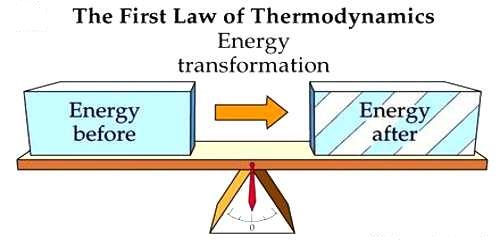# Explanation of the First Law of Thermodynamics

Explanation of the First Law of Thermodynamics

Law: When work is transformed into heat or heat is transformed into work, then work and heat is directly proportional to each other

By applying the first law of thermodynamics a relation between heat, internal energy, and work can be established. Besides, amount of work is known by different thermal processes. Also, an idea is obtained whether the system is absorbing heat or releasing heat. Observe the following explanations:

If due to absorption of dQ amount of heat, the change of internal energy is du and work done is dW, then the first law of thermodynamics can be written in differential form as,

dQ = du + dW …. …. …. (1)

This equation is a special form of the principle of conservation of energy. Equation (1) is a mathematical form of the first law of thermodynamics. It is applicable to all the bodies.

In equation (1), quantities dQ, du and dW may be positive and negative.

(i) dQ will be positive if heat is supplied to the system or heat is absorbed by the system and will be negative it the system knows energy or heat is dissipated to the surroundings from the system.

(ii) du will be positive when the internal energies of the system increases and du will be negative when the internal energy decreases.

(iii) dW will be positive when the system does work in the surroundings and dW will be negative when the surrounding does work on this system.

The first law makes use of the key concepts of internal energy, heat, and system work. It is used extensively in the discussion of heat engines. The standard unit for all these quantities would be the joule, although they are sometimes expressed in calories